Next: Actions Up: FORMALISM Previous: Fluents

## Causality

We shall make assertions of causality by means of a fluentwhere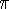is itself a propositional fluent.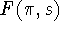asserts that the situation s will be followed (after an unspecified time) by a situation that satisfies the fluent. We may use F to assert that if a person is out in the rain he will get wet, by writing: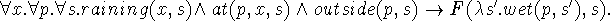Suppressing explicit mention of situations gives: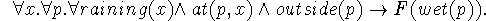In this case suppressing situations simplifies the statement.

F can also be used to express physical laws. Consider the law of falling bodies which is often written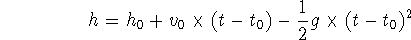together with some prose identifying the variables. Since we need a formal system for machine reasoning we cannot have any prose. Therefore, we write: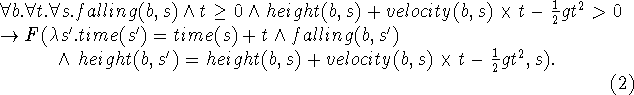Suppressing explicit mention of situations in this case requires the introduction of real auxiliary quantities v, h and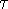so that the sentence takes the following form: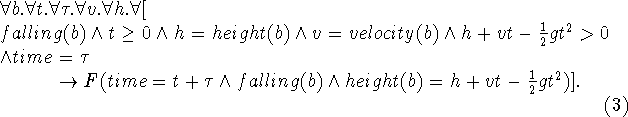There has to be a convention (or declarations) so that it is determined that height(b), velocity(b) and time are fluents, whereas t, v,and h denote ordinary real numbers.as introduced here corresponds to A. N. Prior's (1957, 1968) expression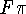.

The use of situation variables is analogous to the use of time-instants in the calculi of world-states which Prior (1968) calls U-T calculi. Prior provides many interesting correspondences between his U-T calculi and various axiomatizations of the modal tense-logics (that is, using this F-operator: see part 5). However, the situation calculus is richer than any of the tense-logics Prior considers.

Besides F he introduces three other operators which we also find useful; we thus have:

1.. For some situation s' in the future of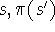holds.

2.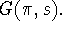For all situations s' in the future ofholds.

3.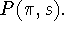For some situations s' in the past ofholds.

4.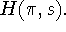For all situations s' in the past ofholds.

It seems also useful to define a situational fluent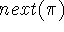as the next situation s' in the future of s for which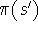holds. If there is no such situation, that is, if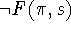, then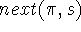is considered undefined. For example, we may translate the sentence `By the time John gets home, Henry will be home too' as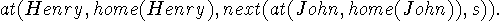Also the phrase `when John gets home' translates into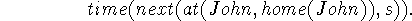Thoughwill never actually be computed since situations are too rich to be specified completely, the values of fluents applied towill be computed.Next: Actions Up: FORMALISM Previous: Fluents

John McCarthy
Mon Apr 29 19:20:41 PDT 1996